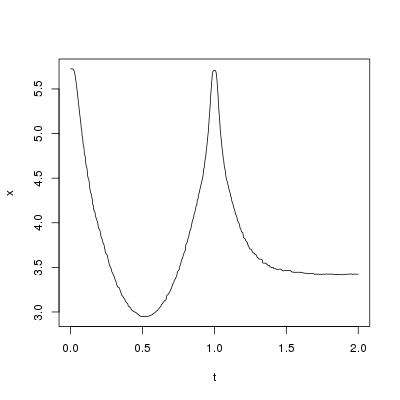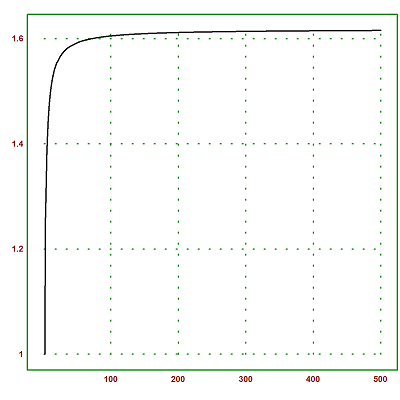# Difference between Arithmetic and Geometric Sequence

Arithmetic and Geometric series helps the mathematicians solve a range of complex problems. Both series and sequence have very similar definitions in the field of mathematics as the end result is a set of infinite or finite elements. Students are advised to learn and understand the differences and similarities between these two terms to make sure they apply these concepts appropriately. Both arithmetic series and geometric series can be infinite or finite as explained before. Furthermore, the number of terms can be easily counted if the series is finite.

Progression has the tendency to be positive infinity for negative common difference. Positive common difference on the other hand gives rise to negative infinity. The formula, developed by Indian astronomer and mathematician Aryabhata, is required to compute the sum of the series in mathematics is given below:

Sn = n/2 (a1+ an ) = n/2 [2a1 + (n-1)d] As explained before, the sum can be either finite or infinite depending on the numbers of terms included in the equation.

If the quotient of the successive numbers is a constant value then that series is known as a geometric series. Because of the variety of problems it can solve and its properties, geometric series is often considered as the most important series formulated. The formula used to determine the sum of terms for this type of series is given below:

Sn = a(1-rn) / (1-r); where Sn is the sum of the series and r is the ratio which categorises the behaviour of the series.

Properties of geometric series can discovered by using the relationship Sn = ar + ar2 + ar3 +⋯+ arn = ∑ni=1 ari.

Geometric series has a variety of applications in the field of engineering, physical sciences and economics.

### Instructions

• 1

Arithmetic series:

The sum of all elements inside a progression is known as a series. If the difference between two elements in a sequence of numbers is a constant then it is an arithmetic series.

Image Courtesy:  sieste.wordpress.com• 2

Geometric series:

Similarly, if the difference between any two numbers in a sequence is a constant quotient then the series is termed as a geometric series.

Image Courtesy:  euler.rene-grothmann.de•